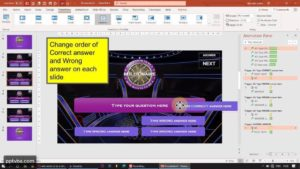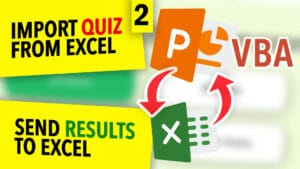PowerPoint Templates & Games

# How to Make a Timer in PowerPoint VBA

In this module, we will be creating a countdown timer in Microsoft PowerPoint using Macros in Visual Basic Applications. Unlike other modules where VBA is not used, we do not have to type every single number and add animations.

VBA Macros focus on automating your work in the least amount of time taken to increase efficiency for your projects.

This PPT Countdown can be embedded in variety of project, modules and PowerPoint Games. You may download this module and code for free and make your presentations more interactive.

## Schematics of PowerPoint Countdown

``````Dim time As Date
time = Now() ``````

The output for Now() is the current time and date.

In our coding, we increase the current time by “count” seconds, which is 30 seconds in our code.
We add “count” seconds using the following code:

``````Dim count As Integer
count = 30 'assuming 30 seconds'
time = DateAdd("s", count, time) ``````

I shall refer to this new time in which the 30 seconds is added as the new future time.

We then wait for the current time to catch up to the new future time.
Meanwhile we use the Do Until Function to update the “countdown” shape.

### Customise the format of PPT Countdown Timer

The following are the syntax for various formats of displaying time in VBA:

Use the format “ss” if you want only the seconds to be visible.

Use the format “nn:ss” if you want only the minutes and seconds to be visible.

Normally, mm and nn are interchangeable when hh is present. However, when hh is not present, mm stands for months and nn stands for minutes.

Use the format “hh:mm:ss”

## How the Countdown works:

We have two main variables:

1. Current Time + “count” seconds (new future time)
2. Current Time

In the beginning we take the current time and add the “count” seconds and store it in time variable. The time variable thus contains the new future time.

We then wait for the current time to catch up to the new future time and until that happens we update the “countdown” shape with the difference of new future time and current time.

## MsgBox Pop-Up Notification when the countdown gets over

If our new future time is surpassed by the current time, we can easily add a Message Box pop-up with an If-Then function. Instead of a Message Box, you can also play a sound effect or redirect the presentation to a certain slide.

Insert this code within the Do-Until Loop.

``````If time < Now() Then
MsgBox "Time Up!"
End If ``````

## Customising Countdown Value within SlideShow Mode.

If you want to change the coutdown value and customise it without touching the code, we can add an ActiveX Element Textbox with the name “TBSeconds” in our slide. The user can type inside “TBSeconds” and its value will be used in place of “count” seconds.

We can read the numerical entered inside “TBSeconds” with the following code snippet:

``````'and instead of assigning count = 30 we can assign the following:'
count = ActivePresentation.Slides(1).Shapes("TBSeconds").OLEFormat.Object.Value ``````

You can also have a shape outside the slide and change its text instead, we can then have read the text inside the “timelimit” shape:

``count = ActivePresentation.Slides(1).Shapes("timelimit").TextFrame.TextRange ``

## Countdown Timer in PowerPoint VBA

``````Sub countdown()

Dim time As Date
time = Now()

Dim count As Integer
count = 30 'assuming 30 seconds'

Do Until time < Now()
DoEvents
ActivePresentation.Slides(1).Shapes("countdown").TextFrame.TextRange = Format((time - Now()), "hh:mm:ss")
Loop

End Sub ``````

## How to countdown to a specific date or time?

``````Sub countdown()

Dim time As Date
'change the date and time within the brackets'
time = DateSerial(2021, 10, 13) + TimeSerial(0, 0, 0)

Do Until time < Now()
DoEvents
ActivePresentation.Slides(1).Shapes("countdown").TextFrame.TextRange = DateDiff("d", Now(), time) & " Days " & Format((time - Now()), "hh:mm:ss")
Loop

End Sub ``````

Instead of using DateAdd() to add seconds or minutes or hours. We are using DateSerial() and TimeSerial() to set a particular time on a particular date to which it should countdown.

## Countup Timer in PowerPoint VBA

``````Sub countup()

Dim time1 As Date, time2 as Date
time1 = Now()
time2 = Now()

Dim count As Integer
count = 30 'assuming 30 seconds'

Do Until time2 < Now()
DoEvents
ActivePresentation.Slides(1).Shapes("countdown").TextFrame.TextRange = Format((Now() - time1), "hh:mm:ss")
Loop

End Sub ``````

# Countdown timer in all slides of powerpoint presentation

Now if we need to embed the same countdown timer throughout multiple PowerPoint slides which will correspond to the countdown, i.e if there is a timer for 30 seconds and you go to the next slide with 15 seconds remaining, the next slide should have the timer and should resume from 15 seconds only. It doesn’t matter after how many seconds you decide to go to the next slide or again the previous slide.

This PowerPoint Countdown Timer will be present throughout the Slide Show and will have all the features mentioned above in this website.

To accomplish this, we would need to add a For Loop.

Using the For Loop in PowerPoint VBA, all the slides in the range of i (which is 1 to 10) will be updated until the current time passes the new future time.

``````Sub countdown()

Dim time As Date
time = Now()

Dim count As Integer
count = ActivePresentation.Slides(1).Shapes("timelimit").TextFrame.TextRange

Do Until time < Now()

DoEvents

For i = 1 To 5
ActivePresentation.Slides(i).Shapes("countdown").TextFrame.TextRange = Format((time - Now()), "ss")
Next i

If time < Now() Then
For i = 1 To 5
ActivePresentation.Slides(i).Shapes("countdown").TextFrame.TextRange = "Time up!"
Next i
ActivePresentation.SlideShowWindow.View.GotoSlide (6)
End If

Loop

End Sub ``````## PowerPoint Quiz Game with Multiple Correct Answers

Select multiple correct answers in a PowerPoint Quiz Game using...## Create “Who Wants To Be A Millionaire” PowerPoint Game

Here, we will be using Microsoft PowerPoint to make an...## How to make Interactive Colouring Book Game in PowerPoint

Learn to make a PowerPoint Quiz Game using Trigger Animations....## Interactive True-or-False QUIZ GAME in PowerPoint

Learn to make a PowerPoint Quiz Game using Trigger Animations....## How to Shuffle PowerPoint Slides in a Random Order

If you want all the slides of a particular range...## Populate A PowerPoint Quiz From Excel With VBA

Import Questions & Answers of a Microsoft PowerPoint Multiple-Choice Quiz...## PowerPoint Educational Game – Free Classroom Chatbot

This PowerPoint Educational Game - Classroom Chatbot can ask questions...